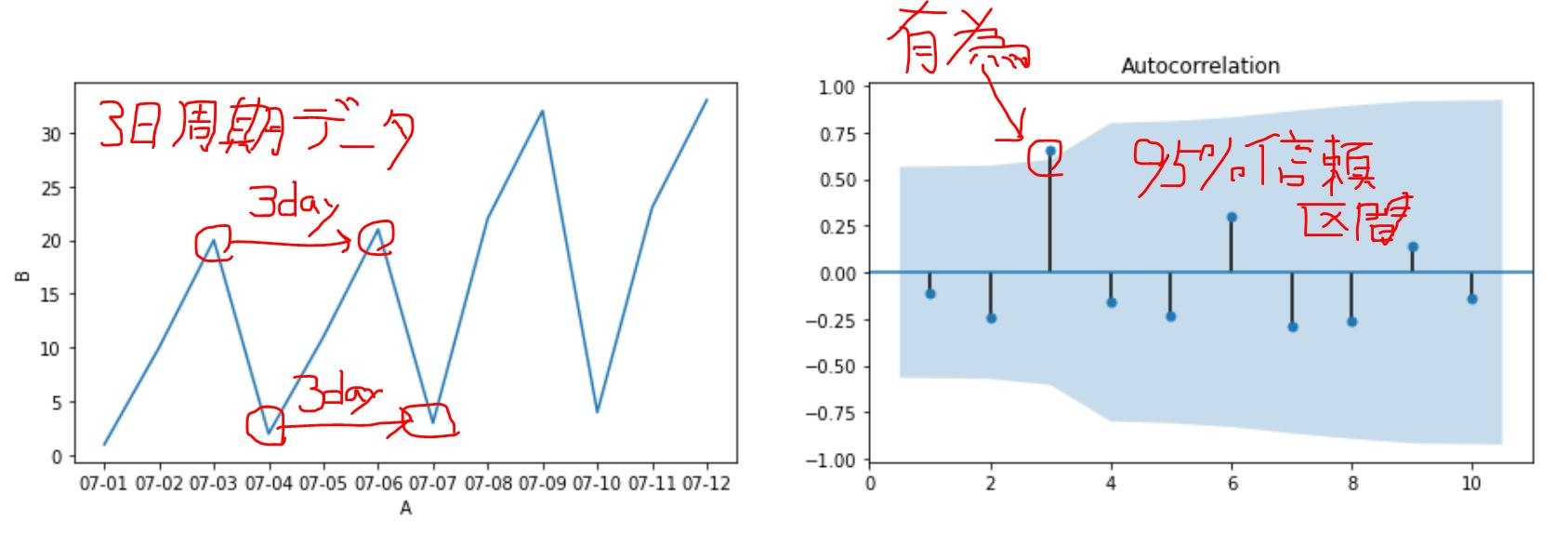# [PYTHON] How to calculate the autocorrelation coefficient

Explain how to calculate the autocorrelation coefficient with python.

# 2 methods

Automatically calculate the autocorrelation coefficient of data with a 3-day cycle. (Use plot_acf)

#### `sample.py`

``````
#Define data.
from statsmodels.graphics.tsaplots import plot_acf
import pandas as pd
import numpy as np

dat = [
['07-01',1],
['07-02',10],
['07-03',20],
['07-04',2],
['07-05',11],
['07-06',21],
['07-07',3],
['07-08',22],
['07-09',32],
['07-10',4],
['07-11',23],
['07-12',33],
]

#Store dat in DataFrame type variable df.
df = pd.DataFrame(dat,columns=["A","B"])

print("Show df","\n",df)

fig, ax = plt.subplots(ncols=2, figsize=(15, 4))
sns.lineplot(x="A", y="B", data=df,ax=ax)
plot_acf(df["B"].dropna(), lags=10, zero=False,ax=ax) #When calculating the autocorrelation, it cannot be calculated correctly if there is NA.
``````